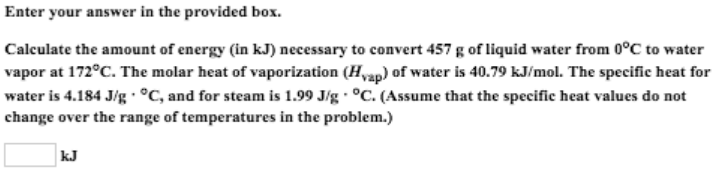# Problem: Enter your answer in the provided box.Calculate the amount of energy (in kJ) necessary to convert 457 g of liquid water from 0°C to water vapor at 172°C. The molar heat of vaporization (Hvap) of water is 40.79 kJ/mol. The specific heat for water is 4.184 J/g • °C, and for steam is 1.99 J/g • °C. (Assume that the specific heat values do not change over the range of temperatures in the problem.)

###### FREE Expert Solution
90% (116 ratings)###### Problem Details

Enter your answer in the provided box.

Calculate the amount of energy (in kJ) necessary to convert 457 g of liquid water from 0°C to water vapor at 172°C. The molar heat of vaporization (Hvap) of water is 40.79 kJ/mol. The specific heat for water is 4.184 J/g • °C, and for steam is 1.99 J/g • °C. (Assume that the specific heat values do not change over the range of temperatures in the problem.)Frequently Asked Questions

What scientific concept do you need to know in order to solve this problem?

Our tutors have indicated that to solve this problem you will need to apply the Heating and Cooling Curves concept. You can view video lessons to learn Heating and Cooling Curves. Or if you need more Heating and Cooling Curves practice, you can also practice Heating and Cooling Curves practice problems.

What professor is this problem relevant for?

Based on our data, we think this problem is relevant for Professor Wilk's class at Columbus State Community College.